Next: 4.5.3.13.1 Scalar Coefficients for Up: 4.5.3 PROMIS-NT Model Classes Previous: 4.5.3.12 Quantity Initialization and

### 4.5.3.13 Coefficients for Segment Models

For each coefficient in (4.1) - (4.2) a separate MDL Model class type is defined to allow for the automatic recognition of confusions in the diffusion model setup by the runtime type recognition system of the Algorithm Library. The naming convention for these Model classes is to prefix the coefficient name written in ASCII characters with CoeffModel_''. Thus the resulting parent models for the volume model coefficients are CoeffModel_alpha, CoeffModel_a, CoeffModel_b, CoeffModel_c, CoeffModel_d, and CoeffModel_gamma.

The values of the dependent variables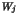are represented by Parameters with the according MDL name MdlQuantity of the affected quantity. The task of the coefficient models is to compute the value of the resulting coefficient and its derivatives to the dependent quantities as a function of the simulation time, the process temperature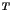, the spatial coordinates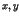of a single grid point, and the values of all involved quantities. Further the derivative to the potential quantity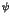has to be computed if the potential is used in the computation of the coefficient value. For that purpose the coefficient models are evaluated once for every grid point of the segment and time step of the transient simulation loop.

Table 4.19 lists the Interface Parameters which can be used for the evaluation of all kinds of segment coefficient models. Special mentioning is due to the Interface Parameters Cown and CownName. CownName contains the literal MDL name of the hereafter called column quantity'' which is the quantity associated with the equation with the indexin (4.1) - (4.2). Cown supplies the according quantity value and is therefore linked to the Parameter describing the respective quantity.

Table 4.19: Parameters common to all segment coefficient Models
Name Type Description
time double
 the simulation time in [s]
T double
 the lattice temperature in [K]
x double
 x coordinate of the actual grid point in [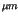]
y double
 y coordinate of the actual grid point in []
Cnet double
 the net doping concentration [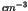]
psi double
 the electrostatic potential [V] or the value of the quantity specified by using the PotentialQuantity parameter described in Section 4.5.3.7.
Cown double
 value of the column quantity
CownName MdlString
 MDL name of the column quantity
MdlQuantity double
 value of the quantity MdlQuantity

It should be noted that the default values of all coefficients and all derivatives are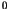. Thus derivatives and coefficients withvalues need not to be computed explicitly.Next: 4.5.3.13.1 Scalar Coefficients for Up: 4.5.3 PROMIS-NT Model Classes Previous: 4.5.3.12 Quantity Initialization and
Robert Mlekus
1999-11-14